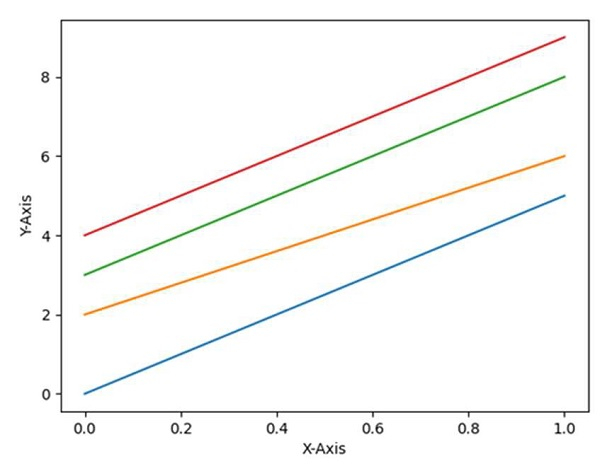# How to pick a new color for each plotted line within a figure in matplotlib?

To pick a new color for each plotted line within a figure, use the following steps −

• Setup X-axis and Y-axis labels for the diagram.

• Set the current .rc parameters. For axes facecolor, the group is axes.

• Use a cycler to set the color for the group of lines. The color list consists of ‘r’ for red, ‘g’ for green, ‘b’ for blue, and ‘y’ for yellow.

• Cycler class helps to create a new Cycler object from a single positional argument, a pair of positional arguments, or the combination of keyword arguments.

• Plot the number of lines with different colors.

• Use plt.show() to show the figure.

## Example

import matplotlib.pyplot as plt
from cycler import cycler

plt.ylabel("Y-axis ")
plt.xlabel("X-axis ")

plt.rc('axes', prop_cycle=(cycler('color', ['r', 'g', 'b', 'y'])))

plt.plot([0, 5])
plt.plot([2, 6])
plt.plot([3, 8])
plt.plot([4, 9])
plt.show()

## Output